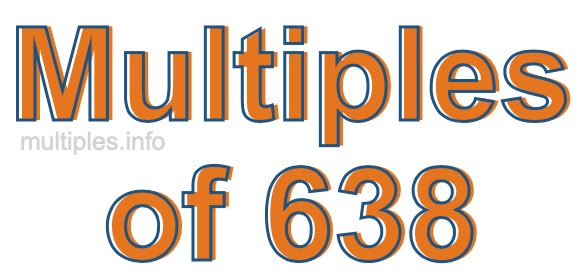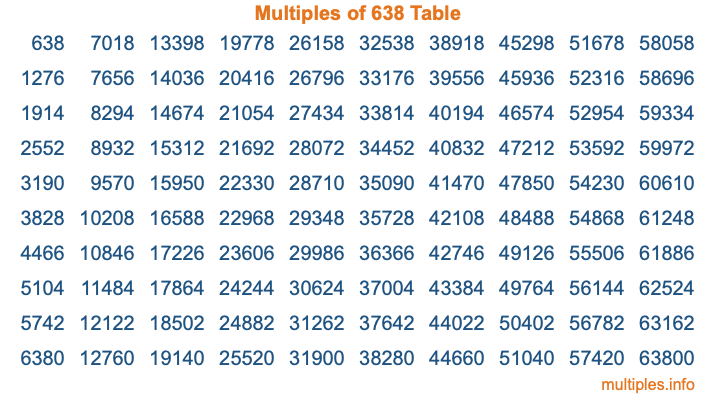Multiples of 638Welcome to the Multiples of 638 page. Here we will first teach you everything you will ever need to know about the multiples of 638, and then give you a study guide summary of everything we taught you to make sure you remember it all. Use this page to look up facts and learn information about the multiples of 638. This page will make you a multiples of six hundred thirty-eight expert!

Definition of Multiples of 638
Multiples of 638 are all the numbers that when divided by 638 equal an integer. Each of the multiples of 638 are called a multiple. A multiple of 638 is created by multiplying 638 by an integer.

Therefore, to create a list of multiples of 638, you start with 1 multiplied by 638, then 2 multiplied by 638, then 3 multiplied by 638, and so on for as long as you want. Thus, the list of the first five multiples of 638 is 638, 1276, 1914, 2552, and 3190. To see a larger list of multiples of 638, see the printable image of Multiples of 638 further down on this page. We also have a category where you can choose any nth multiple of 638.

Multiples of 638 Checker
The Multiples of 638 Checker below checks to see if any number of your choice is a multiple of 638. In other words, it checks to see if there is any number (integer) that when multiplied by 638 will equal your number. To do that, we divide your number by 638. If the the quotient is an integer, then your number is a multiple of 638.

Is  a multiple of 638?

Least Common Multiple of 638 and ...
A Least Common Multiple (LCM) is the lowest multiple that two or more numbers have in common. This is also called the smallest common multiple or lowest common multiple and is useful to know when you are adding our subtracting fractions. Enter one or more numbers below (638 is already entered) to find the LCM.

Check out our LCM Calculator if you need more details about the Least Common Multiple or if you need the LCM for different numbers for adding and subtraction fractions.

nth Multiple of 638
As we stated above, 638 is the first multiple of 638, 1276 is the second multiple of 638, 1914 is the third multiple of 638, and so on. Enter a number below to find the nth multiple of 638.

th multiple of 638

Multiples of 638 vs Factors of 638
638 is a multiple of 638 and a factor of 638, but that is where the similarities end. All postive multiples of 638 are 638 or greater than 638. All positive factors of 638 are 638 or less than 638.

Below is the beginning list of multiples of 638 and the factors of 638 so you can compare:

Multiples of 638: 638, 1276, 1914, 2552, 3190, etc.

Factors of 638: 1, 2, 11, 22, 29, 58, 319, 638

As you can see, the multiples of 638 are all the numbers that you can divide by 638 to get a whole number. The factors of 638, on the other hand, are all the whole numbers that you can multiply by another whole number to get 638.

It's also interesting to note that if a number (x) is a factor of 638, then 638 will also be a multiple of that number (x).

Multiples of 638 vs Divisors of 638
The divisors of 638 are all the integers that 638 can be divided by evenly. Below is a list of the divisors of 638.

Divisors of 638: 1, 2, 11, 22, 29, 58, 319, 638

The interesting thing to note here is that if you take any multiple of 638 and divide it by a divisor of 638, you will see that the quotient is an integer.

Multiples of 638 Table
Below is an image of the first 100 multiples of 638 in a table. The table is in chronological order, column by column. The first column has the first ten multiples of 638, the second column has the next ten multiples of 638, and so on.The Multiples of 638 Table is also referred to as the 638 Times Table or Times Table of 638. You are welcome to print out our table for your studies.

Negative Multiples of 638
Although not often discussed or needed in math, it is worth mentioning that you can make a list of negative multiples of 638 by multiplying 638 by -1, then by -2, then by -3, and so on, to get the following list of negative multiples of 638:

-638, -1276, -1914, -2552, -3190, etc.

Multiples of 638 Summary
Below is a summary of important Multiples of 638 facts that we have discussed on this page. To retain the knowledge on this page, we recommend that you read through the summary and explain to yourself or a study partner why they hold true.

There are an infinite number of multiples of 638.

A multiple of 638 divided by 638 will equal a whole number.

638 divided by a factor of 638 equals a divisor of 638.

The nth multiple of 638 is n times 638.

The largest factor of 638 is equal to the first positive multiple of 638.

638 is a multiple of every factor of 638.

638 is a multiple of 638.

A multiple of 638 divided by a divisor of 638 equals an integer.

638 divided by a divisor of 638 equals a factor of 638.

Any integer times 638 will equal a multiple of 638.

Multiples of a Number
Here you can get the multiples of another number, all with the same attention to detail as we did for multiples of 638 on this page.

Multiples of
Multiples of 639
Did you find our page about multiples of six hundred thirty-eight educational? Do you want more knowledge? Check out the multiples of the next number on our list!